# SOF - IMO PDF Sample Papers for Class 2

Resources:

Class 2 sample paper & practice questions for International Mathematics Olympiad (IMO) level 1 are given below. Syllabus for level 1 is also mentioned for these exams. You can refer these sample paper & quiz for preparing for the exam.Q.1 Q.2 Q.3 Q.4 Q.5 Q.6 Q.7 Q.8 Q.9 Q.10
 Q.1 How many different numbers are shown below?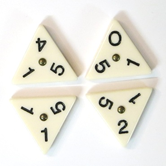a) 4 b) 6 c) 5 d) 12
 Q.2 Which three numbers among the following options have a sum of 789? a) 70 + 80 + 9 b) 700 + 800 + 9 c) 699 + 79 + 11 d) 700 + 89 + 9
 Q.3 Which of the following image represents semi-circle? a)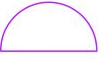b)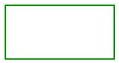c)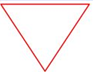d)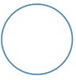Q.4 What is the minimum amount required to buy all the three items?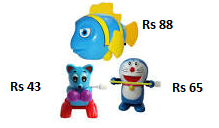a) Rs. 190 b) Rs. 196 c) Rs. 206 d) Rs. 186
 Q.5 Which circle contains the smallest number of parts? a)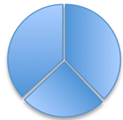b)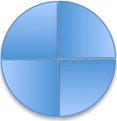c)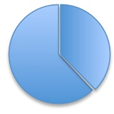d)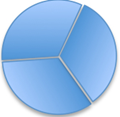Q.6 Find the odd one out. a) Delhi b) Mumbai c) New York d) Kolkata
 Q.7 Find the missing weight to balance the scale.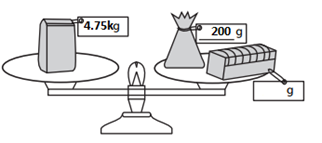a) 3550 kg b) 455 kg c) 4.55 kg d) 4550 kg
 Q.8 What date was on Thursday after the second Friday of the month?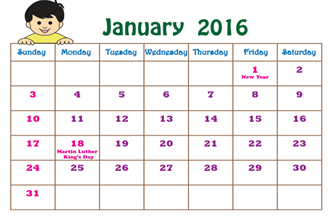a) 15th January b) 8th January c) 14th January d) 13th January
 Q.9 Find the missing figure and complete the pattern given below: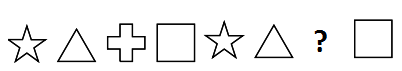a)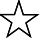b)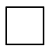c)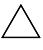d)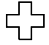Q.10 Find the middle of the ruler.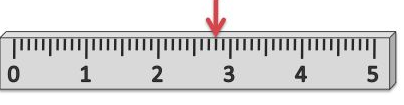a) Exactly below the red arrow b) To the left of the red arrow c) To the right of the red arrow d) Can not findSample PDF of SOF - International Mathematics Olympiad (IMO) PDF Sample Papers for Class 2: